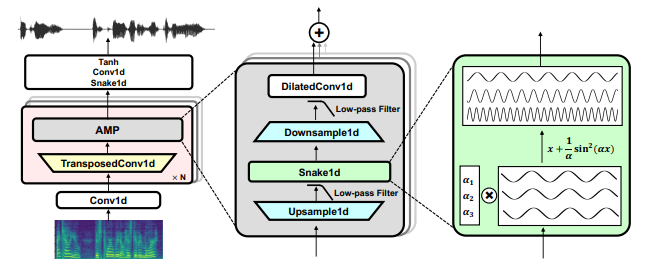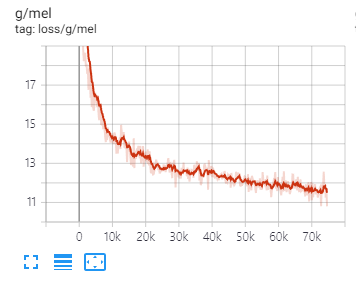# BigVGAN: A Universal Neural Vocoder with Large-Scale TrainingIn this repository, I try to implement BigVGAN (specifically BigVGAN-base model) [Paper] [Demo].

## Pre-requisites

1. Pytorch >=3.9 and torchaudio >= 0.9

## Training Exmaple

```# VCTK
python preprocess.py

python train_bigvgan_vocoder.py -c configs/vctk_bigvgan.json -m bigvgan```

## 2022-06-12

• Current ver has some redundant parts in some modules (e.g., data_utils have some TTS module. Ignore it plz)

## BigVGAN vs HiFi-GAN

1. Leaky Relu –> x + (1/a)*sin^2(ax)

2. MRF –> AMP block

• Up –> Low-pass filter –> Snake1D –> Down –> Low-pass filter
• I need to review this module. I used torchaudio to implement up/downsampling with low-pass filter. I used the rolloff value of 0.25 in the T.resample but I’m not sure this value is equal to sr/(2*m) where m is 2.
• torchaudio > 0.9 is needed to use the rolloff parameter for anti-aliasing in T.resample
• There are some issues of STFT function in pytorch of 3.9 (When using mixed precision, waveform need to be changed to float before stft )
1. MSD –> MRD (Univnet discriminator) UnivNet unofficial github

2. max_fre 8000 –> 12,000 for universal vocoder (sr: 24,000)

• We use the sampling rate of 22,050 and linear spectrogram for input speech.

## Low-pass filter

• pytorch extension ver with scipy??? StarGAN3
• torchaudio ver (0.9 >=) can use Kaiser window and rolloff (cutoff frequency)

Torchaudio tutorial

torchaudio.transforms is much faster than torchaudio.functional when resampling multiple waveforms using the same paramters.

```# beta
m = 2
n = 6
f_h = 0.6/m
A = 2.285*((m*n)/2 - 1)*math.pi*4*f_h+7.95
beta = 0.1102*(A-8.7)
4.663800127934911```

## Results (~ing)Audio

I train the BigVGAN-base model with batch size of 64 (using two A100 GPU) and an initial learning rate of 2 × 10−4

(In original paper, BigVGAN (large model) uses batch size of 32 and an initial learning rate of 1 × 10−4 to avoid an early training collapse)

For the BigVGAN-base model, I have not yet experienced an early training collapse with batch size of 64 and an initial learning rate of 2 × 10−4

## Automatic Mixed Precision (AMP)

The original paper may not use the AMP during training but this implementation includes AMP. Hence, the results may be different in original setting.

For training with AMP, I change the dtype of representation to float for torchaudio resampling (Need to be changed for precise transformation).

View Github